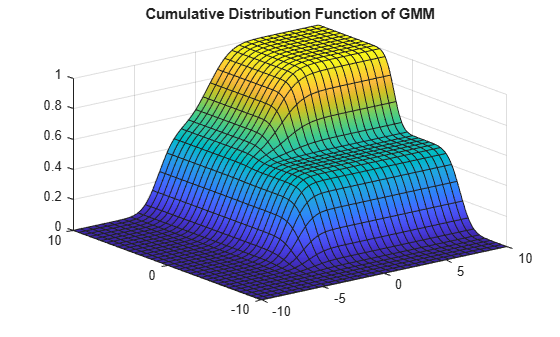# Create Gaussian Mixture Model

This example shows how to create a known, or fully specified, Gaussian mixture model (GMM) object using `gmdistribution` and by specifying component means, covariances, and mixture proportions. To create a GMM object by fitting data to a GMM, see Fit Gaussian Mixture Model to Data.

Specify the component means, covariances, and mixing proportions for a two-component mixture of bivariate Gaussian distributions.

```mu = [1 2;-3 -5]; % Means sigma = cat(3,[2 0;0 .5],[1 0;0 1]); % Covariances p = ones(1,2)/2; % Mixing proportions```

The rows of `mu` correspond to the component mean vectors, and the pages of `sigma`, `sigma(:,;,J)`, correspond to the component covariance matrices.

Create a GMM object using `gmdistribution`.

`gm = gmdistribution(mu,sigma,p);`

Display the properties of the GMM.

`properties(gm)`
```Properties for class gmdistribution: NumVariables DistributionName NumComponents ComponentProportion SharedCovariance NumIterations RegularizationValue NegativeLogLikelihood CovarianceType mu Sigma AIC BIC Converged ProbabilityTolerance ```

For a description of the properties, see `gmdistribution`. To access the value of a property, use dot notation. For example, access the number of variables of each GMM component.

`dimension = gm.NumVariables`
```dimension = 2 ```

Visualize the probability density function (pdf) of the GMM using `pdf` and the MATLAB® function `fsurf`.

```gmPDF = @(x,y) arrayfun(@(x0,y0) pdf(gm,[x0 y0]),x,y); fsurf(gmPDF,[-10 10]) title('Probability Density Function of GMM');```Visualize the cumulative distribution function (cdf) of the GMM using `cdf` and f`surf`.

```gmCDF = @(x,y) arrayfun(@(x0,y0) cdf(gm,[x0 y0]),x,y); fsurf(gmCDF,[-10 10]) title('Cumulative Distribution Function of GMM');```